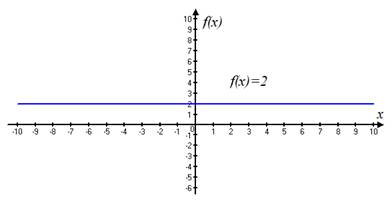# Sketch the graph of the function f ( x ) = 2 .### Precalculus: Mathematics for Calcu...

6th Edition
Stewart + 5 others
Publisher: Cengage Learning
ISBN: 9780840068071### Precalculus: Mathematics for Calcu...

6th Edition
Stewart + 5 others
Publisher: Cengage Learning
ISBN: 9780840068071

#### Solutions

Chapter 2.2, Problem 5E
To determine

## Sketch the graph of the function f(x)=2 .

Expert Solution

f(x) The graph of the given function is:### Explanation of Solution

Given information:consider the function f(x)=2 .

Calculation:

The function f(x)=b , where b is a given number, is called a constant function because all its values are the same number, namely, b. Its graph is the horizontal line y=b

The graphs of the constant function f(x)=2 as shown below:### Have a homework question?

Subscribe to bartleby learn! Ask subject matter experts 30 homework questions each month. Plus, you’ll have access to millions of step-by-step textbook answers!